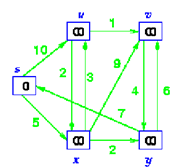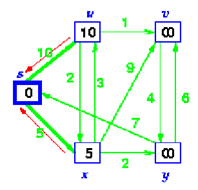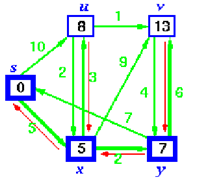## Operation of algorithm, Data Structure & Algorithms

Assignment Help:

Operation of Algorithm

The following sequence of diagrams shows the operation of Dijkstra's Algorithm. The bold vertices show the vertex to which shortest path has been find out.

Step 1:

Initialize the graph, all of the vertices have infinite costs except the source vertex that has zero costStep 2

From all the adjacent vertices, select the closest vertex to the source s.

As we initialized d[s] through 0, it's s. (illustrated in bold circle)

Relax all vertices adjacent to s, that means u & x

Update vertices u & x by 10 & 5 as the distance from s.Step 3:

Select the nearest vertex, x. Relax all of vertices adjacent to x

Update predecessors for u, v & y. Predecessor of x = s

Predecessor of v = x ,s

Predecessor of y = x ,sStep 4:

Now y is the closest vertex. Add it to S.

Relax v and adjust its predecessor.Step 5:Step 6:

The predecessor list now defines the shortest path from each node to s.

#### Arrays, Data array A has data series from 1,000,000 to 1 with step size 1, ...

Data array A has data series from 1,000,000 to 1 with step size 1, which is in perfect decreasing order. Data array B has data series from 1 to 1,000,000, which is in random order.

#### The threaded binary tree, By changing the NULL lines in a binary tree to th...

By changing the NULL lines in a binary tree to the special links called threads, it is possible to execute traversal, insertion and deletion without using either a stack or recursi

#### Compare two functions, Comp are two functions n 2    and  2 n  / 4...

Comp are two functions n 2    and  2 n  / 4  for distinct values of n.   Determine When s ec on d function b ec om es l a r g er th an f i r st functi

#### Complete trees, This is a k-ary position tree wherein all levels are filled...

This is a k-ary position tree wherein all levels are filled from left to right. There are a number of specialized trees. They are binary trees, AVL-trees, binary search trees, 2

#### Depth first search, DEPTH FIRST SEARCH (DFS) The approach adopted into ...

DEPTH FIRST SEARCH (DFS) The approach adopted into depth first search is to search deeper whenever possible. This algorithm frequently searches deeper through visiting unvisite

#### Simplifying assumptions of wire frame representation, Simplifying Assumptio...

Simplifying Assumptions of wire frame representation Neglect colour - consider Intensity: For now we shall forget about colour and restrict our discussion just to the intensi

#### Indexed sequential files, Indexed Sequential Files An index is inserted...

Indexed Sequential Files An index is inserted to the sequential file to provide random access. An overflow area required to be maintained to permit insertion in sequence. I

#### Define stack lifo, A stack is a last in, first out (LIFO) abstract data typ...

A stack is a last in, first out (LIFO) abstract data type and sequential data structure. A stack may have any abstract data type as a component, but is characterized by two fundame

#### #input restricted DEQUE, #why all the 4 operations i.e. insertion n del...

#why all the 4 operations i.e. insertion n deletion from rear end and front end is valid in input restricted DEQUE

#### Data flow diagrams, How to construct a data flow diagram for a college assi...

How to construct a data flow diagram for a college assignment and marking systemA